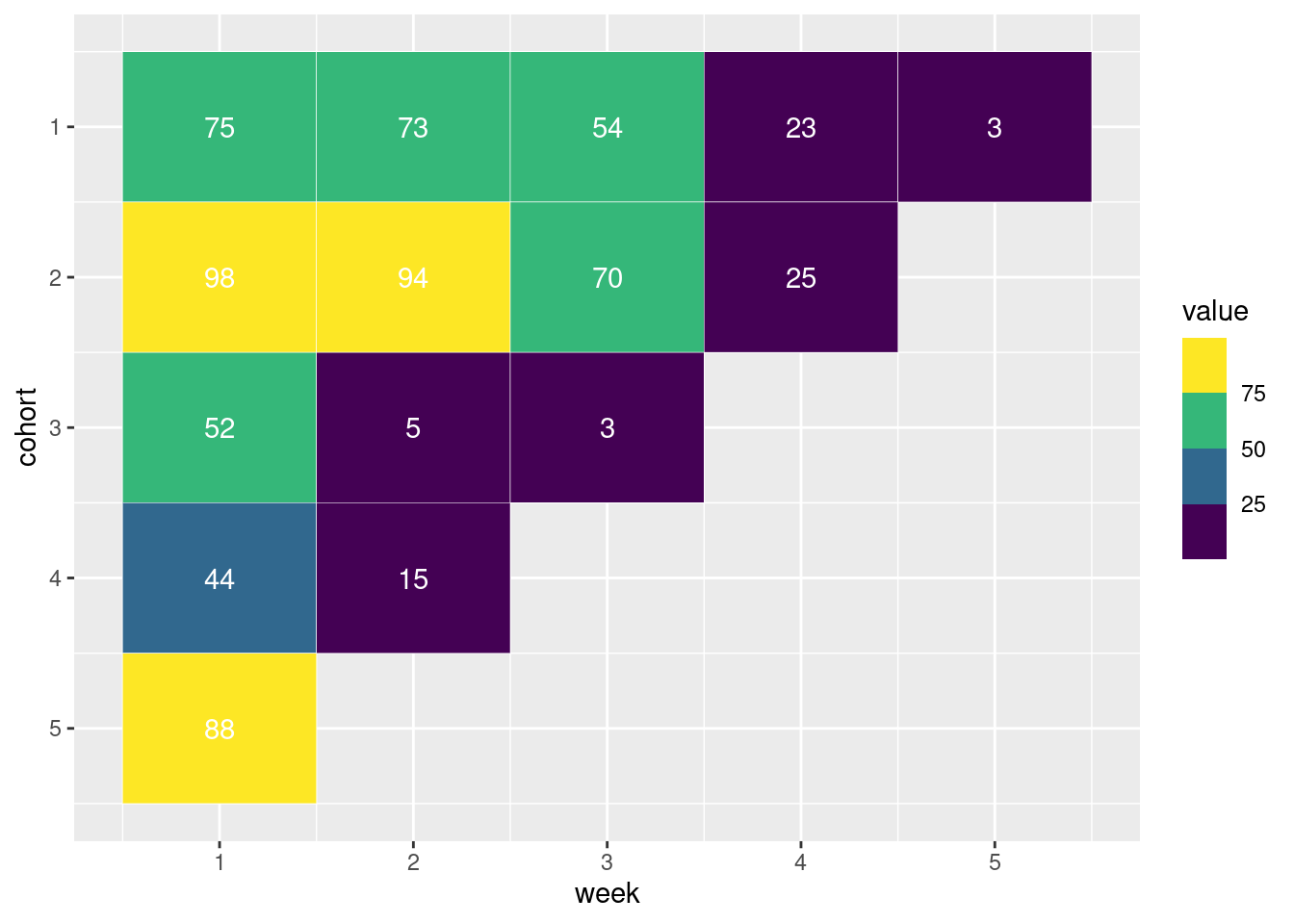## 12.23 留存图

cohort <- data.frame(
cohort = rep(1:5, times = 5:1),
week = c(1:5, 1:4, 1:3, 1:2, 1),
value = c(
75, 73, 54, 23, 3,
98, 94, 70, 25,
52, 5, 3,
44, 15,
88
)
)

ggplot(cohort, aes(x = week, y = cohort, fill = value)) +
geom_tile(color = "white") +
geom_text(aes(label = value), color = "white") +
scale_y_reverse() +
scale_fill_binned(type = "viridis")